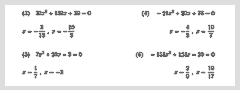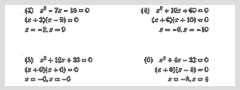# Home | Mathematics | Quadratic equationQuadratic equation parametersSecond-Degree EquationSolve it by factoringAlgebra, Equation, Solving